# Abstracts

Results of practical importance had been discarded testing formulated hypothesis with the aid of statistical analysis of experimental data because of the power of the utilized test. This study compares the power of two Bonferroni's Modified and one Sidak's Modified tests with known tests analyzing 1200 simulated experiments. All differences of means were obtained in relation to the mean of the adopted control to guarantee parametrical magnitude of mean differences. Student's test (type I comparisonwise error) and Waller-Duncan's (Bayesian error) showed the highest percentage of significative differences, followed by Duncan's, BM2, SiM, BM1, DunnettU's, SiN, BN, Dunnettu's, SNK's, REGWF's, REGWQ's, Tukey's, Sidak's and Bonferroni's tests. For differences equal to zero, Student's and Waller-Duncan's test exhibit 5% frequency of rejection of the null hypothesis, in accordance the nominal error I adopted (alpha = 0.05). All other tests had values below 0.05, generally ranging on 0.01 to 0.02 or less. Depending of the number of zero differences and considering the type I experimentwise error I, Student's, Waller-Duncan's and Duncan's tests showed crescent values of errors (> 0.05), proportional to the number of null differences included in the experiment; all other tests exhibit showed of type I experimentwise error < 0.05, most nearing 0.01-0.02 or less. Efficiency of the three "Modified Tests" was close to DunnettU's test, but higher than the other testes of type I experimentwise error nature (MEER).

statistical tests; efficiency of tests; multiples comparisons

testes estatísticos; eficiência dos testes; comparações múltiplas

STATISTICS

Bonferroni's and Sidak's modified tests

Teste modificado de Bonferroni e Sidak

Armando ConaginI; Décio BarbinII, * * Corresponding author < debarbin@esalq.usp.br>

IInstituto Agronômico de Campinas, C.P. 28 - 13020-902 - Campinas, SP - Brasil

IIUSP/ESALQ - Depto. de Ciências Exatas, C.P. 9 - 13418-900 - Piracicaba, SP - Brasil

ABSTRACT

Results of practical importance had been discarded testing formulated hypothesis with the aid of statistical analysis of experimental data because of the power of the utilized test. This study compares the power of two Bonferroni's Modified and one Sidak's Modified tests with known tests analyzing 1200 simulated experiments. All differences of means were obtained in relation to the mean of the adopted control to guarantee parametrical magnitude of mean differences. Student's test (type I comparisonwise error) and Waller-Duncan's (Bayesian error) showed the highest percentage of significative differences, followed by Duncan's, BM2, SiM, BM1, DunnettU's, SiN, BN, Dunnettu's, SNK's, REGWF's, REGWQ's, Tukey's, Sidak's and Bonferroni's tests. For differences equal to zero, Student's and Waller-Duncan's test exhibit 5% frequency of rejection of the null hypothesis, in accordance the nominal error I adopted (a = 0.05). All other tests had values below 0.05, generally ranging on 0.01 to 0.02 or less. Depending of the number of zero differences and considering the type I experimentwise error I, Student's, Waller-Duncan's and Duncan's tests showed crescent values of errors (> 0.05), proportional to the number of null differences included in the experiment; all other tests exhibit showed of type I experimentwise error < 0.05, most nearing 0.01-0.02 or less. Efficiency of the three "Modified Tests" was close to DunnettU's test, but higher than the other testes of type I experimentwise error nature (MEER).

Key words: statistical tests, efficiency of tests, multiples comparisons

RESUMO

Palavras-chave: testes estatísticos, eficiência dos testes, comparações múltiplas

INTRODUCTION

To expand the knowledge in different areas of the experimental sciences, experiments are set up to test hypothesis that best explain the phenomena under investigation. Statistical methods are used to design experiments, to choose group of treatments, to perform analyses of variance, to do statistical tests and to estimate parameters. All those methods provide ways to prove or not the formulated hypothesis.

The tests more frequently used for comparison of treatments (means) are Student's, Duncan's, Student-Newman-Keul's (SNK), Tukey's and Dunnett's. Bonferroni's, Sidak's and Waller-Duncan's testes are used less than often.

Studies have been conducted to evaluate both the power of these tests and the different types of errors involved. The evaluation of the power of each test is obtained through the calculus of the percentage of significative differences in which the probability of error of type I  the rejection of the null hypothesis  adopted is a = 0.05 or 0.01.

More recently, errors type I were classified in type I comparisonwise error and type I experimentwise error, the type I experimentwise error under condition of the general null hypothesis, and the type I experimentwise error under condition of partial hypothesis and maximum experimentwise error rate (MEER) type (SAS, 2004), considering both situations. Both unilateral and bilateral Student's tests and Waller-Duncan's test guarantee protection to comparisonwise errors; SNK's enables protection to general experimentwise error, and Tukey's, Bonferroni's, Sidak's, REGWF's, REGWQ's and Dunnett's answers to errors of MEER type.

Evaluations of the efficiency of various statistical tests were done by Gabriel (1964), Carmer & Swanson (1971; 1973), O'Neill & Wetherill (1971), Boardman & Moffitt (1971), Bernardson (1975), Chew (1977), Petersen (1977), Thomas (1974), Hochberg & Tanhame (1987), and others. The efficiency of different tests more frequently utilized in Brazil was also evaluated by Perecin & Barbosa (1988), Conagin (1998), Conagin et al. (1999), Dos Santos (2000), and Conagin & Pimentel-Gomes (2004). The objective of this paper is evaluating the comparative efficiency of the more frequently utilized tests, and the behavior of the comparisonwise and experimentwise type errors, varying the magnitude of differences, number of treatments, number of replications, and number of degrees of freedom of the residual. The paper also introduces a Bonferroni's Modified and a Sidak's Modified test.

MATERIAL AND METHODS

The power of Student's unilateral (Tl), bilateral (T2), Duncan's (D), Waller-Duncan's (W), Student-Newman-Keul's (SNK), Tukey's (Tu), Bonferroni's (B), Sidak's (Si), Dunnett's (Du) and (DU), Modified Bonferroni's (BM and BM2), Modified Sidak's (SiM), REGWF and REGWQ, Gin SAS's default method was tested; in the Modified tests, a = 0.05. Three groups  G1, G2 and G3  included the tests with three, four, six, and eight replications. A discussion on the modifications of the Bonferroni's and Sidak's follow.

Bonferroni's Test

The Bonferroni's test may be used to calculate confidence interval and comparison of means. In this paper, only differences were studied. Means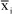andof two treatments with ri and rj replications, differ significantly if:where df is the number of degrees of freedom of QM Res,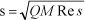, and t(ab , df) is the value of the t-distribution with probability ab and df degrees of freedom. If ri = rj = r, then.

If a given experiment has t treatments with r replications, and a = 0.05 is the global probability for k comparisons, in which H0: T1 = T2 = ... = Tt = 0 is the general null hypothesis, then, for all comparisons between pairs of means,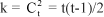, and in this case.

If H0 is true, the probability of not rejecting any difference is (1-aB)k. If the global probability adopted is a = 0.05 or 0.01 (for t treatments), the probability of rejecting the general H0 will be:and considering only the first two terms, a » 1 [1 - kaB]. Then aB = a/k.

The calculus of the significative difference between two means is done applying Student's test with aB and df degrees of freedom. The test assures a = 0.05 for all tests, and the error is of the MEER experimentwise type.

Modified Bonferroni's Test (BM)

Modified Bonferroni's test was proposed by Conagin (1999). The Student's t for the test shall be obtained in Student's Tables, with degree of freedom of the residual and a' probability level, in which a' = a(1 + P), and ordinarily a = 0.05 or 0.01. The calculus of P is presented bellow. In the analysis of variance, the test of H0 is obtained by the test F0 = QMTreat/QMresidual, in which there are t treatments and r replications (e.g. in randomized blocks design). The critical value of F is Fc, with (t  1) and (r  1) (t  1) degrees of freedom, for a = 0.05 or 0.01.

The parametric model is Xij = M + Ti + Bj + Eij, in which i = 1, 2, ... t, and j = 1, 2,..., r. The expected values are:If the general H0 is true, T1 = T2 = ... = Tt = 0. For the sampling model (experimental),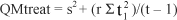being s2 the QMresidual. In the Analysis of Variance of the experiment:Due to the size of experimental error some treatments of real nature are not significative.

If F0 > Fc, H0 is rejected. Then, there should be one or more treatments te¹ 0. The parameter of non-centrality of the F distribution, if Ha (alternative hypothesis) is true, is l (Winer et al., 1991); this value is:For a given experiment,Then.

The evaluation of P(F0) and P(Fc), if Ha is true, may be obtained by PROB F Function of SAS (2004). For fixed t and r:P = P(F0)  P(FC) is then defined. This is the probability represented by the area between F0 and FC in the F non-central. If FC is fixed, the area increases if F0 increases. The area is originated and due to treatments that are far from(general mean) and includes the treatment significant different of.

With a'/k, smaller values than the t value when H0 is true are obtained. If t'< t, the efficiency of the test will be increased.

The corresponding a' for this situation is defined as a'= a(1+P), and then aBM = a'/k. In this case, the t value of the Table of Student test for a' value produces t'< t, where t' is the value of the Table according to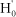(where the new hypothesis have smaller number of treatments in which, being l < t).

The modified Bonferroni's test uses the t Student test with probability a', and then aBM = a'/k.

Values shown in Tables 1, 2, 5 , and 6 , use the PROBF Function to calculate P. But since Group G2 includes 25 treatments, ordinarily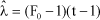produces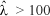, and then, PROBF Function do not calculate the P(F0) and P(FC). In this case, calculated Tables (Conagin, 2001) for a = 0.05, t, r and F0/FC included in the cells the corresponding P value are used. If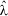> 100 and F0/FC > 7, an proximate value for P using F0/FC = 7 and= 100 is used. The justificative is that if F0/FC>, 7 the area (and then P) should be greater than F0/FC = 7, and then adopted P is a conservative value.

Second Modified Bonferroni's Test (BM2)

If in the Analysis of variance F0 > FC (general H0 rejected), there should be a treatment parametrically different of zero. It is possible to evaluate a by â and use it to calculate BM2, as follows.

If F0 > FC the ANOVA is performed and the significant differences between two means by the Student's test (comparisonwise type I error) are calculated. The â number of significant differences is used as an estimate of the true number of parametrical differences between treatments. This happens because, in general, experiments are performed to evaluate responses of new "treatments", supposedly superior to treatments ordinarily used (especially in agronomy, animal science and veterinary medicine, medical, biological, and industrial research, and some other areas in which a new or new treatments are supposed to be better, in some way, than currently used treatments). The â number of significant differences should be â < a. Due to the size of experimental error, some treatments actually different from zero but of small values, result in non-significant differences, and then â is, probably, a conservative estimate of the true a value (â < a).

The probability modified Bonferroni's (BM2) is then: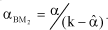The modified Bonferroni's test behaves similarly to tests of the type MEER, as can be possibly seen in the columns 0% (exp) of Tables 1, 2, 3, 4, 5 , and 6 . Their frequencies are very much alike the corresponding Dunnett's frequency and it is well known that Dunnett's test is of MEER type.

Sidak's Test (Si)

The Sidak's test uses a probability as and, similarly to Bonferroni's, use the Student t test with level as and df degrees of freedom of the residual. The as value is calculated from: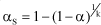in which as is the global probability and k is the number of comparisons between means.

Ordinarily, if the interest lies in all comparisons between two means. To compare each treatment with a control, k = t  1; this type appear as SiN in the tables. The value as is greater than aB and then the corresponding tS is smaller than tB. So Sidak's test is a little more efficient than Bonferroni's. For instance, for a = 0.05, k = 10, as = 0.0512, and aB = 0.05. Therefore, tS is smaller than tB.

Modified Sidak's Test (SiM)

Similarly to Second Bonferroni's Modified (BM2), after performing an ANOVA of a given experiment, if F0 > FC (H0 general hypothesis rejected), significant differences are calculated by the t test; the number of significant differences â is an estimate of the number of all the actual differences a. Then: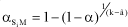This test tends to be a little more effective than the correspondent Bonferroni's (BM2), because aSiM > aBM2. The calculus of significative differences in relation to a control uses t test with k=(t-1)-â. If interest lies in all differences between two means, k=(t(t-1)/2)-â. The behavior of the application of Modified Sidak's test can be evaluated in Tables 1, 2, 3, 4, 5 , and 6 .

Power of the different tests in the groups G1, G2 and G3

To evaluate the power of Student's t test T2 (bilateral), Duncan's, SNK's, Tukey's, Bonferroni's, Dunnett's, BN's, BM's, BM2's, SiN's and SiM's, simulations of two hundred experiments, r = 3 replications and considering diferences of 0.4; 0.3; 0.25; 0.15; 0.1; 0.05 and 0.0 (both cp and exp); and one hundred experiments, r = 6 replications, groups G1 (12 treatments) and G2 (25 treatments), were analyzed. For both groups, CV = 10%. In group G2, a Waller-Duncan's test (W) was included. The "default criterion" (SAS, 2004) was used for the ANOVA and for the Modified Bonferroni's and Sidak's tests, calculus of significant differences (a = 0.05) were done separately by the authors.

In group G3 (Tables 5 and 6 ), parametrical differences were 0.3, 0.2, 0.15, 0.1, 0.05, and 0.0 (both cp and exp), for r = 4 (400 experiments) and r = 8 (200 experiments). Once again "default criterion" was used for the ANOVA tests and a = 0.05 for the modified Bonferroni's and Sidak's test. Data this group were also submitted to REGWF's, REGWQ's, Student's unilateral (T1), Dunnett's unilateral (DU) tests, and Sidak's basic (Si) test, in which k = t(t-1)/2.

For large differences (i.e. 0.4, 0.35, 0.3, and 0.25) on G1 and G2 (Tables 1 and 2), and G2 alone (Tables 3 and 4), tests showed high power, with little differences among them. Differences in the power of the various tests increased for differences 0.2, 0.15, and 0.1 to values often and more ordinarily obtained in the research data analysis. In groups G1 and G2, power of tests were, in order, Waller-Duncan (W), Student bilateral (T2), Duncan (D), SNK, SiM, BM, BM2, SiN, BN, Dunnett, Tukey and Bonferrooni.

Regarding error of type I comparisonwise [column 0% (cp)], Student's and Waller-Duncan's tests showed values a » 0.05, the nominal error adopted. The other tests showed a < 0.05, most of them around 0.01 or smaller. The error I per experiment (column 0% exp; Tables 3 and 4) of Student's (T2), Waller-Duncan's and Duncan's tests were much higher than 0.05; other tests showed errors smaller than 0.05, most nearing 0.01, or smaller.

In the group G3 (t = 8; n = 4 and 8; CV = 10%), there were differences of efficiency among tests for values of 0.3 or less (Table 5 ). In the range of 0.1-0.2, Student's unilateral (T1) was slightly superiority to Waller-Duncan's (W) and Student's bilateral (T2), that increases when r = 8 (Table 6 ). The overall order of efficiency registered was: T1, T2, W, D, SiM, DU, BM, BM2, SiN, BN, REGWF, REGWQ, Tu, Si and B.

Regarding errors of type I comparisonwise [column 0% (cp)], W, T1, T2, and D, showed a = 0.05, the nominal error adopted. The other tests showed a < 0.05, most nearing 0.01. For errors of type I experimentwise [column o% (exp)], T1, T2, W, and D, showed a values well above 0.05; the other tests showed a values smaller than 0.05, most nearing 0.01 or less. The power of all the tests increased when the number of replications increased. It is therefore easier to compare values in the Tables 1, 3, and 5 , with the corresponding values in Tables 2, 4, and 6 , respectively.

Accepted December 28, 2005

• BERNARDSON, C.S. Type I error rates when multiple comparison procedures follows a significant F test of Anova. Biometrics, v.31, p.337-340, 1975.
• BOARDMAN, T.J.; MOFFITT, D.R. Graphical Monte Carlo type I error rates for multiple comparison procedures. Biometrics, v.27, p.738-744, 1971.
• CARMER, S.G.; SWANSON, M.R. Detection of differences between means, a Monte Carlo study of five multiple comparison procedure. Agronomy Journal, v.63, p.940-945, 1971.
• CARMER, S.G.; SWANSON, M.R. Evaluation of ten multiple comparison procedures by Monte Carlo methods. Journal of American Statistical Association, v.68, p.66-74, 1973.
• CHEW, V. Comparisons among treatment means in an analysis of variance Beltsville: USDA, 1977. 69p. (Bulletin, H6).
• CONAGIN, A. Discriminative power of Modified Bonferroni's Test. Revista de Agricultura, v.73, p.31-46, 1998.
• CONAGIN, A. Discriminative power of the Modified Bonferroni's Test under general and partial null hypothesis. Revista de Agricultura, v.74, p.117-126, 1999.
• CONAGIN, A. Tables for the calculation of the probability to be used in the Modified Bonferroni's Test. Revista de Agricultura, v.76, p.71-83, 2001.
• CONAGIN, A.; PIMENTEL-GOMES, F. Escolha adequada dos testes estatísticos para comparações múltiplas. Revista de Agricultura, v.79, p.288-295, 2004.
• CONAGIN, A.; IGUE, T.; NAGAI, V. Poder discriminativo de diferentes testes de médias Campinas: Instituto Agronômico, 1999. (Boletim Científico, 44).
• DOS SANTOS, C. Novas alternativas de testes de agrupamento avaliadas por meio de simulação Monte Carlo. Lavras: UFLA, 2000. 85p. (Dissertação M.S.).
• GABRIEL, K.R. A procedure for treating the homogeneity of all set of means in analysis of variance. Biometrics, v.20, p.459-477, 1964.
• HOCHEBERG, Y.; TAMHAME, A.C. Multiple comparisons procedures New York: John Wiley & Sons, 1987. 450p.
• O'NEILL, R.; WETERILL, G.B. The present state of multiple comparison methods. Journal of the Royal Statistical Society, v.33, p.218-250, 1971.
• PERECIN, D.; BARBOSA, J.C. Uma avaliação de seis procedimentos para comparações múltiplas. Revista de Matemática e Estatística, v.6, p.95-103, 1988.
• PETERSEN, R.G. Use and misuse of multiple comparison procedures. Agronomy Journal, v.69, p.205-208, 1977.
• SAS INSTITUTE. SAS/STAT 9.1: User's Guide. Cary: SAS Institute Inc., 2004. 5136p.
• THOMAS, D.H. Error rates in multiple comparisons among means results of a simulation exercise. Applied Statistics, v.23, p.284-294, 1974.
• WALLER, R.A.; DUNCAN, D.B. A Bayes rule for the symmetric comparison problem. Journal of the American Statistical Association, v.67, p.253-255, 1972.
• WINER, B.J.; BROWN, D.R.; MICHELS, K.M. Statistical principles in experimental designs 3.ed. New York: McGraw-Hill, 1991. 1057p.
••• *
Corresponding author <

# Publication Dates

• Publication in this collection
22 Feb 2006
• Date of issue
Feb 2006

• Accepted
28 Dec 2005